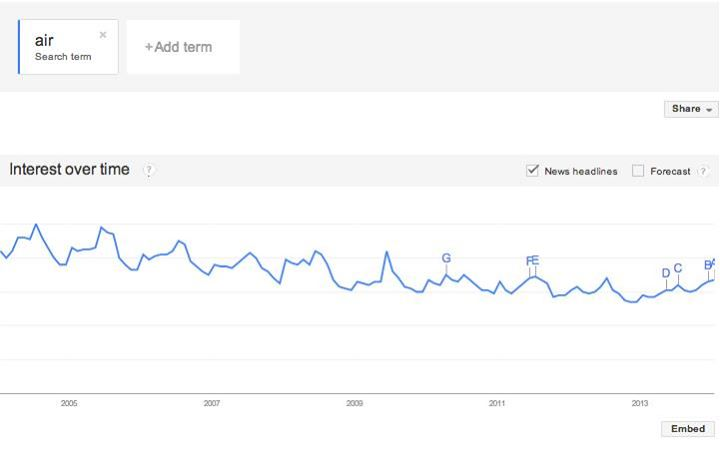# Statistics - (Factor Variable|Qualitative Predictor)

A factor is a qualitative explanatory variable.

Each factor has two or more levels, i.e., different values of the factor.

Combinations of factor levels are called treatments.

Example:

• character variable,
• or a string variable

## Modelling a factor

We can't put categorical predictors into a regression analysis function. We need to make it a numeric variable in some way. That's where dummy coding comes in.

### Two levels

Example with gender which has two levels (male or female) We create a new variable

$$X = \left\{\begin{array}{ll} 1 & \text{ if the person is a male} \\ 0 & \text{ if the person is a female} \end{array}\right.$$

Resulting model:

$$Y_i = B_0 + B_1 X_i + \epsilon_i = \left\{\begin{array}{ll} B_0 + X_i + \epsilon_i & \text{ if the ith person is a male} \\ B_0 + \epsilon_i & \text{ if the ith person is a female} \end{array}\right.$$

### More than two

With more than two levels, we create additional dummy variables.

For example, for a colour variable with three levels (blue, red, green), we create two dummy variables.

$$\begin{array}{lll} X_1 & = & \left\{\begin{array}{ll} 1 & \text{ if the colour is blue} \\ 0 & \text{ if the colour is not blue} \end{array}\right. \\ X_2 & = & \left\{\begin{array}{ll} 1 & \text{ if the colour is red} \\ 0 & \text{ if the colour is not red} \end{array}\right. \\ \end{array}$$

Then both of these variables can be used in the regression equation, in order to obtain the following model:

$$Y_i = B_0 + B_1 X_{i1} + B_2 X_{i2} + \epsilon_i = \left\{\begin{array}{ll} B_0 + B_1 + \epsilon_i & \text{ if the ith colour is blue} \\ B_0 + B_2 + \epsilon_i & \text{ if the ith colour is a red} \\ B_0 + \epsilon_i & \text{ if the ith colour is a green } \\ \end{array}\right.$$

There will always be one fewer dummy variable than the number of levels. The level with no dummy variable Green in this example is known as the baseline.

Discover More(Machine|Statistical) Learning - (Predictor|Feature|Regressor|Characteristic) - (Independent|Explanatory) Variable (X)

A Independent variable is a variable used in supervised analysis in order to predict an outcome variable. It's also known as: Predictor Input variable, Regressors, Explanatory variable, CovariateCovariates...Data Mining - Problem

A page the problem definition in data Type of target: nominal or quantitative Type of target class: binomial of multiclass Number of parameters: Type of (predictor|features): nominal or numeric....R - (Dummy Code|Categorical Variable) in Regression

Dummy codes in order to handle Categorical Variable With a categorical predictor, dummy codes to represent the nominal variable as numeric using the function C (for contrasts) contrasts...R - K-Nearest Neighbors (KNN) Analysis

in R. where: k is number of neighbours to be considered. train is the training set c1 is the factor of the training set with the true target test is the test set The knn function is...Statistics - (Level|Label)

The levels of an variable are the number of distinct value that the variable contains. See also A level or label is therefore one distinct value for the variable. In a experiment, the combinations...Statistics - Analysis of variance (Anova)

Anova is just a special case of multiple regression. There're many forms of ANOVA. It's a very common procedure in basic statistics. Anova is more Appropriate when: there's true independent variable...Statistics - Confounding (factor|variable) - (Confound|Confounder)

In statistics, a confounding variable (also confounding factor, a confound, or confounder) is an extraneous variable in a statistical model that correlates (directly or inversely) with both the dependent...Statistics - Correlation does not imply causation

Correlation does not imply causation In the late 1940s, public health experts recommended that people stop eating ice cream as part of an anti-polio diet. It turned out however that there was...Statistics - Dummy (Coding|Variable) - One-hot-encoding (OHE)

Dummy coding is: a classic way to transform nominal into numerical values. a system to code categorical predictors in a regression analysis A system to code categorical predictors in a regression...Statistics - Factorial Anova

A factorial ANOVA is done when the independent variables are categorical. By adding a second independent variable, we are entering in factorial ANOVA. N Independent Variables (IVs). Variables that...